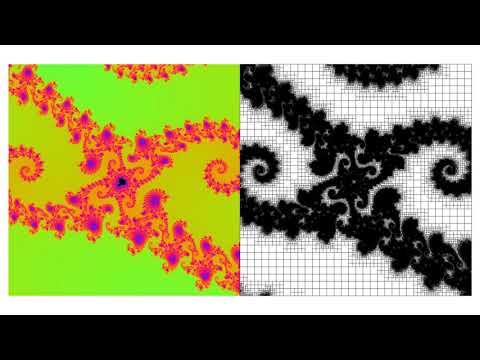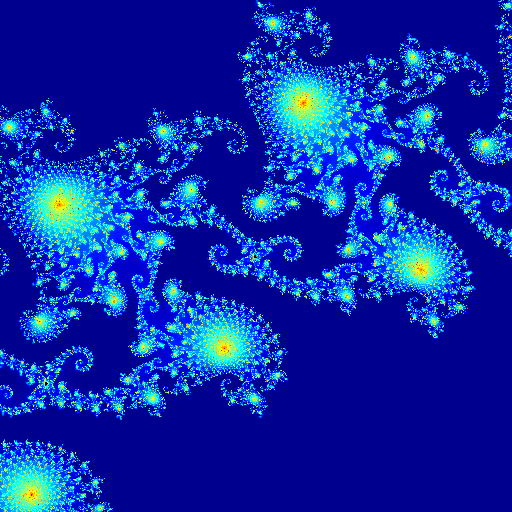# A visualization of the Mandelbrotset using adaptive grids.

For a given complex number \mathcal{c} one can define the sequence of numbers z_{n} indexed with n as,

\displaystyle z_{n+1}=z_n^2+\mathcal{c},

starting from z_0=\mathcal{c}. It can be shown that the sequence diverges in an absolute sense if z_nz_n^* > 2. The mandelbrot set can be visualized using color coding for the amount of iterations it takes to get z_n such that z_nz_n^* \geq 2. Furthermore, there are sequenses that do not diverge (eg. the sequence corresponding to c=-1). Therefore we choose to stop checking for divergence after n_{max}=2000 iterations.

A quadtree grid will be used, for the output we use standard functions supplemented with a costum color map named doublerainbow, after it’s colorful palette.

#include "grid/quadtree.h"
#include "utils.h"
#include "view.h"

void doublerainbow (double cmap[NCMAP]){
for (int i = 0; i < NCMAP; i++){
cmap[i]=sq(sin((double)i*M_PI/64.));
cmap[i]=sq(sin(((double)i+20.)*M_PI/64.));
cmap[i]=sq(sin(((double)i+40.)*M_PI/64.));
}//Black saturation
cmap[NCMAP-1]=0;
cmap[NCMAP-1]=0;
cmap[NCMAP-1]=0;
}

We zoom in on a pre-specified location. Here xy and yz are the real and imaginary part of the complex number that specifies the zoom-in location, respectively.

double xz=-0.7447450302052016;
double yz=0.1216630821053122;

The visualization uses 2 scalar fields and a intermediate/dummy field. The number of iterations untill convergence are stored in the cc scalar field. However, for visualization purposes we visualize the logarithm of that number. So the color-coded field that has a linear mapping to the color bar is stored in c. Furtheremore, for maximum visual statisfaction, we mask the cells that do not divergece within jmax iterations, their locations are stored in the m field.

scalar c[],cc[],m[];
double jmax=2000.;

A grid resolution is set that corresponds to the resolution of the movie.

int maxlevel = 9;
double mm,avg,it,f,ag;
int i;

The zoom-in corresponds to a 2^{1040/40} = 67108864 times magnificaton.

int end = 520;
double perit = 40;

We define a function that calculates how many iterations it takes before the aforementioned sequence diverges. If it does not diverge within jmax iterations, it will return jmax. Also we track the total number of iterations made for each snapshot and store the result in ag.

double determine_iterations(double x,double y){
double j=0.;
double xx=xz+(x/f);
double yy=yz+(y/f);
double a=xx;
double b=yy;
double c;
double ab=(sq(a)+sq(b));
while (j<jmax && ab < 4){
c=(a*a)-(b*b)+xx;// Real part
b=(2*a*b)+yy; //Imag part
a=c;
j+=1.;
ab=((a*a)+(b*b));
}
ag+=j; //Track the total number of iterations required for all cells.
return (double) j;
}

int main(){

The color coded field c is calculated via the cc field each iteration, and does not rely on the values of the old iteration.

  m.refine=refine_injection; //cheap refinement

We set-up a 4 \times 4 box. Large enough to initially show the region of interest of the Mandelbrot set, open a file for the statistics and initialize a grid at the maximum resolution.

  X0=-2.;
Y0=-2.;
L0=4.;
FILE * fp2 = fopen("nja.dat","w");
init_grid(1<<maxlevel);
for (i = 0;i<=end;i++){

At th start of the main zoom-in loop, the total number of iterations made by the solver is reset and f is defined as the magnification factor, its value increases each iteration.

    ag=0;
f = pow(2,(double)i/perit);

Since f is a global variable, we can calculate the number of iterations untill divergence for each cell. Notice that the \{x,y\} coordinates are stretched in the determine_iterations() function itself.

    foreach(reduction(+:ag))
cc[]=determine_iterations(x,y);

We approximate the total number of iterations that would have been required to do the calculations on an 2^{2\text{maxlevel}} grid and store the result in fg. The field with linear colorcoding c is calculated and furthermore, we set the mask field m.

    double fg=0;
double l10 = log(10.);
foreach(reduction(+:fg)){
c[]=log(cc[]+1)/l10;
fg+=pow(4.,(maxlevel-level))*cc[];
m[]=nodata;
m[]=-1.;
}

The result for the check of adaptivity is printed to a file so that we may assess wheather it was smart enough to have used the grid adaptation strategy.

    fprintf(fp2,"%d\t%g\t%g\t%g\n",i,fg/ag,ag,fg);
fflush(fp2);
fprintf(ferr,"%d\t%g\t%g\t%g\n",i,fg/ag,ag,fg);

For the linear interpolation of the colors we need values at the locations of the ghost points within the grid. Furtheremore we uses bview draw commands to plot the most interesting stuff.

    boundary({c});
clear();
squares("m",min = -1, max =2,map=gray); //display mask
squares("c",map=doublerainbow,min=0,max=3.1,linear= true); // Display colors
begin_translate(x=L0);
cells();
end_translate();
save("c.mp4");
if (i==(end-1))
output_ppm(c,file = "cc2.gif", n=pow(2,maxlevel) ,mask=m, linear=true, min=2, max=3.2);

As a last step, the grid is adapted based on the smoothnes of the color-coded field c. Notice that we have an expression for the refinement criterion for this case! Since the color axis ranges from 0 to 3, and there a are 128 (i.e NCMAP) colours in our pallette, the estimated error in c should not exeed 3/128 because that would correspond to a different color in our visualization. Also note that we only update m so that is does not update c and cc.

    adapt_wavelet({c},(double[]){3./128.},maxlevel,5,{m});
}
}

## Results

We have zoomed-in

This is the movie:

Movie

Most of the time spend for this script is towards the visualization itself, therefore a serious speed-up can be achieved by using hardware accelerated rendering. Therefore, you may view the results from a deeper zoom evaluated with my laptop (taking approx. 45 min.) using 10 levels of refeminement. The video is hosted via Vimeo (click thumbnail), Make sure to select the full resolution option!Last frame of the movie above

We can check if the employed adaptivity algorithm did a good job. We compare the total number of iterations done versus the (approximated) number of iterations required on a regular 512\times512 grid.

set key right bottom box
set xr [ 0:1040]
set xlabel 'Frame number'
set ylabel 'Effort Compession'
plot "nja.dat" using 1:2 w l lw 3 title "'Compression' ratio due to adaptivity"(script)